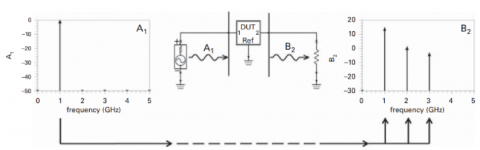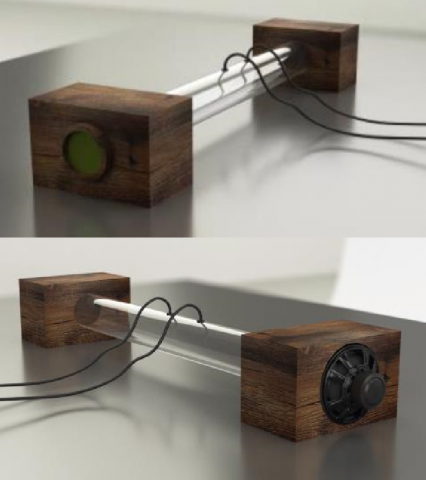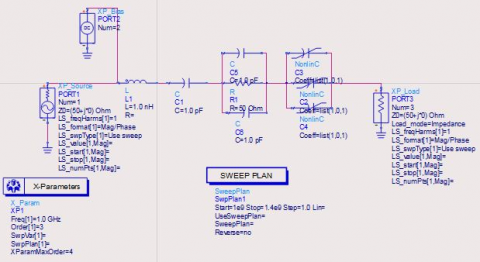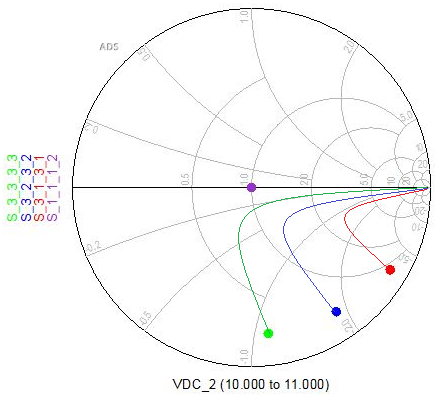# Implementation of X-Parameters Principle for Non-linear Vibroacoustic Membrane Using Two-Port Measurement

Implementation of X-Parameters Principle for Non-linear Vibroacoustic Membrane Using Two-Port Measurement

Reza MoezziJaroslav Hlava Trieu Minh Vu

Institute for Nanomaterials, Advanced Technologies and Innovation, Technical University of Liberec, Studentská 2, 461 17, Liberec, Czech Republic

Faculty of Mechatronics, Informatics and Interdisciplinary Studies, Technical University of Liberec, Studentská 2, 461 17, Liberec, Czech Republic

Department of Electrical Power Engineering and Mechatronics, Tallinn University of Technology, Ehitajate tee 5, 19086, Tallinn, Estonia

Corresponding Author Email:
Reza.Moezzi@tul.cz
Page:
297-301
|
DOI:
https://doi.org/10.18280/ts.360401
16 April 2019
|
Accepted:
29 July 2019
|
Published:
7 October 2019
| Citation

OPEN ACCESS

Abstract:

X-parameters, a powerful solution to the simulation of nonlinear microwave components, can be used for other wave systems with distributed parameters. This paper introduces X-parameters to the acoustic field to deal with highly nonlinear large signals and boundary conditions. Firstly, an elastic membrane was selected to serve as the nonlinear boundary. Then, the equivalent electronic lumped model of the elastic membrane was established based on its nonlinear differential equation (NDE). The X-parameters of the model were determined by poly-harmonic distortion modelling. Then, an electrical analogy of the nonlinear system was established by two port method. The simulation and experimental results show that the properties of the elastic membrane were characterized well by the X-parameters.

Keywords:

X-parameters, poly-harmonic distortion (PHD), S-parameters, lumped model, nonlinear acoustics, scattering matrix

1. Introduction

For the decades, S-parameters, or scattering matrix, have been among the most important of all the foundations of microwave theory and techniques in electronic network analyzing. Graphical methods based around the Smith chart were used to visualize and interpret S-parameters. In acoustics field, the duct system can be explained as a network of uniform straight ducts. The straight ducts are coupled together via different passive elements such as; bends, T-sections, mufflers, etc. When the linear object is investigated, the linear theory can be characterizing the object in term of reflection and absorption coefficient. Åbom  defined the Scattering Matrix of the two-port in linear condition which exactly refers to S-parameters in four-pole electrical network theory. Also, the two-port methodology is applied to characterize the acoustics elements under pure tone or random excitation, an investigation about harmonic interaction effects using two-tone excitations was made by Boden , furthermore the study has been expanded to multi tone in Ref. . Two port S-parameters can be used for linear element, but the nonlinear elements generate other harmonic frequencies under single tone frequency. Bodén  applied Polyharmonic Distortion Modelling to Acoustic Characterization of Perforates which was inspired by Jan Verspect who first investigated describing functions which can better model hard nonlinearities in the frequency domain than the Volterra theory . Beside these investigations, Bellet and Cochelin  was interested to observe Nonlinear Targeted Energy Transfer (TET) phenomena in duct acoustics with nonlinear membrane; they implemented the equivalent mechanical analogy to interpret the occurrence of the TET. Moezzi  attempted to expand the TET concept with applying different boundary conditions in duct acoustics. Almost in all recent research, the S-parameter concept applied to characterize the behavior of the OUT (Object Under Test).

Latest references on application of RF (Radio Frequency) for nonlinear exhibited properties can be seen as follows: Design of hardware for RF dynamic range and distortion was presented by Narayanan, et al. , One port device was designed to simulate the extraction procedure of extraction model for poly harmonic distortion was shown by Martín-Guerrero, et al. . Parameter estimation of nonlinear large-scale systems through stochastic methods and measurement of its dynamic response was designed by Dimitrios and Alexandros . A novel kernel regularized nonlinear GMC model and its application was designed by Ma, et al. . Deconvolution of vibroacoustic images using a simulation model based on a three-dimensional point spread function was presented by Talita, et al. . Complex background suppressing for vibro-acoustography images that map out the acoustic response and acoustic emission was introduced in Ref. . Some complexed signal processing on specific topic with harmonics was designed by Krčmařík, et al. . Design of wireless communication network and database archiving smart systems was presented by Minh, et al. . Sophisticated nonlinear formulas, calculations, and simulations were introduced by Minh, et al. . And the application of AI neural and fuzzy for nonlinear system calculations was presented by Moezzi, et al. .

So, in this paper, we will take into account the concept of X-parameters and simulate the equivalent electronic lumped model of elastic membrane absorber based on its NDE (Nonlinear Differential Equation) in ADS©. The paper will be organized as follows: the first part will give a brief introduction on X-parameters and explain why X-parameters are not reliant on the phase. Then, some experimental results of acoustics nonlinear observation are presented. An electrical analogy of the system with two port method is designed. Experimental and simulations results of this method are presented. Finally, some remarks and conclusion of this paper is given.

2. Methodology

To define the X-parameters, first, it is important to realize that the nonlinear DUT (Device Under Test) performance in large signal field, cannot be described by linear S-parameters. In a simple but important case, it will be confirmed that a DUT description in terms of a nonlinear spectral mapping of signals defined on a harmonic frequency grid overcomes this limitation. The principle of time invariance also is raised to obtain the final form for “single-tone” X-parameters. It can be considered the output of a nonlinear element when a high sinusoidal signal with frequency f1 is applied at port number 1, the output would be an amplified of the sinusoidal input signal and other signals with frequencies which are the harmonics of f1 as shown in Figure 1.

## 1.pngFigure 1. Stimulus and response

The scattered waves from the output port, B2(t), Comprises important energy at harmonic frequencies. Generally, the energy is scattered in the frequency domain on a grid, ωn, defined by the input, ω1

$\omega_{k}=k \omega_{1}$  (1)

The magnitude and the phase of the stimulus construct the complex amplitude of the response at each harmonic at any of the ports which depends on A1,1 (Stimulus). This dependence could be represented in Eq. (2), which is generalized to an arbitrary number of ports, N:

$B_{p, k}=F_{p, k}\left(A_{1,1}\right), p=1,2, \dots, N, n=1,2, \dots, K$  (2)

Eq. (2) shows a nonlinear spectral mapping of the input, A1,1, to the responses of the system, Bp,k.

Another important concept to explain the X-parameters concept is time-invariance, The time-invariance condition imposes some useful properties upon the nonlinear functions Fp,k in Eq. (2).

Assuming the response at port 2 of the DUT, B2(t), due to the input applied at port 1, A1(t), the behavior of the nonlinear DUT is described by an operator O, in Eq. (3).

$b_{2}(t)=O\left[a_{1}(t)\right]$  (3)

If the stimulus is postponed by a time τ, the response necessity be delayed by the same time duration τ, as shown Eq. (4)

$b_{2}(t-\tau)=O\left[a_{1}(t-\tau)\right]$  (4)

In general, The X-parameters for the multi-harmonic inputs would be defined as (5)

$\begin{array}{l}{X_{p, k}^{(F B)}\left(\left|A_{1,1}\right|, A_{1,2} P^{-2}, A_{2,1} P^{-1}, A_{2,2} P^{-2}\right)=} \\ {\frac{F_{p, k}\left(A_{1,1}, A_{1,2}, A_{2,1}, \cdot, A_{2,2}\right)}{p^{k}}}\end{array}$  (5)

which, Am,n are the inputs at port number(m) and harmonic (n), P is defined as

$P=e^{j \cdot p h a s e\left(A_{1,1}\right)}$  (6)

It is concluded that X-parameters are not reliant on the phase of the fundamental due to the multiplication of each harmonic complex-valued argument with the coefficient Pk , which time shifts each harmonic to the moment when the fundamental has a phase of 0o, effectively removing the dependence on the phase of the fundamental .

3. Experimental Acoustics, Non-linear Observation

In this study, the non-linear phenomena are studied in a specific configuration. The primary linear system is represented by a straight duct with total length of 2m and inner diameter of 0.1m where the acoustic waves, generated by an external loudspeaker, propagate in planar linear regime. The essentially non-linear attachment is represented by an elastic, circular membrane. In this scenario, there are two coupling boxes, one between the loudspeaker and the duct and another one between the duct and the membrane as shown in Figure 2.

The acoustical excitation has been provided by a loudspeaker HERTZ EV165 (140 W, 120 mm diameter) in the right-hand side of the rig. The loudspeaker is driven by a software-based signal generator through National Instruments™ NI9269 analog output module and power amplifier (Velleman™ VPA2100MN). The signal acquisition has been performed by a dynamic signal analyzer (National Instruments™ NI NIcDAQ 9174 and NI 9234), controlled by PC based virtual instrument (LabVIEW™).

## 2.pngFigure 2. Rendered picture of experimental setup (right and left view)

The acoustic pressure has been measured by using two flush mounted ¼” pre-polarized pressure microphones (type 40BD G.R.A.S.™, equipped with preamplifiers 26CB G.R.A.S.™). The microphone separation distance is s=300mm. According to the relationship 0.1π<ks<0.8π , the corresponding frequency region reckoned during the measurements ranges from 57 Hz to 457 Hz at the temperature of 20 °C. One of the two microphones (indicated as Mic.2) is located at the midsection of the duct (distance 1m from the end sections). Details about the characteristic material of the membrane are listed in Table 1 and taken from manufacturer datasheet.

Table 1. Membrane properties

 Diameter 100 mm Thickness 0.5 mm Material Latex Pre-stress 20 kPa Young’s Modulus 1 Mpa Poisson’s ratio 0.5

## 3.pngFigure 3. Pressure amplitude at the midsection of the duct (Mic.2) showing the Non-linear behaviour of membrane

For the excitation range examined, the nonlinear behavior occurs from fn2 Hz (92Hz) to fn+5 Hz (97Hz), thus in a quite limited frequency range. Due to the two expansion chambers confining the duct, at the resonance frequency the values of acoustic pressure reached within the duct are higher. Moreover, the minima reached at the occurrence of nonlinear behaviors are at ≈60 Pa. The result is shown in Figure 3.

This figure clearly shows, despite the increasing of loud speaker signal, there is nonlinear behavior and reduction of pressure at Mic2 in certain frequencies. The Figure 4 represents the relation between frequency, amplitude and how both affects the reflection coefficient of the membrane. This is clearly dealing with the definition of nonlinear system which the pressure is not anymore just function of frequency. The reflection coefficient is structured with simultaneous effect of amplitude and frequency together.

## 4.pngFigure 4. Experimental result; Simultaneous effect of frequency and amplitude on reflection coefficient of the membrane

4. Electrical Analogy of the System

To investigate the characterization of membrane the two-port (four-pole) is required, the details are as Figure 5 .

## 5.pngFigure 5. Two-port method to characterize acoustical properties of membrane

The nonlinear differential equation which expresses the transversal displacement of the membrane (q) is obtained by considering the nonlinear plate equation of the Von-Karman type (large displacements, small strains and moderate rotations) taking account of a constant in-plane pre-stress . Because under the pressure applied by the air in the coupling box, the thin visco-elastic circular clamped membrane performs large amplitude oscillations. Typically, the displacement at the center can be about 10 mm, which is 10–30 times larger than the membrane thickness.

The second order, nonlinear differential Eq. (7) express the displacement of the membrane:

$m \ddot{q}+k_{1}[(1+\mu) q+\beta \dot{q}]+k_{3}\left(2 \beta q^{2} \dot{q}+q^{3}\right)=0.5 S_{m} p(t)$  (7)

where, the coefficients k1 and k3, respectively, stand for the linear and nonlinear stiffnesses. The parameter $\mu$  is the ratio between the pre-strain e0 and the (strain) buckling load of the membrane. Other coefficients can be found in same Ref. .

The equivalent differential equation with respect to electrical analogy would be:

$L \ddot{Q}+\frac{1}{c_{1}} Q+\cdot \frac{1}{c_{1}} \cdot R_{1} \dot{Q}+2 R_{1} \frac{1}{c_{3}} Q^{2} \dot{Q}+\frac{1}{c_{3}} Q^{3}=E(t)$  (8)

where, Q is charge of the electrical circuit and dots represent the differentiation in time. L is inductance corresponds to mass of membrane, c1 and c3 are linear and nonlinear capacitors which corresponds to springs, R1 is the linear resistor equivalent to linear damper. E(t) is the AC source voltage corresponds to acoustics pressure applied to membrane.

5. Simulation and Results

For the simulation of the system, the advanced design system software (ADS) is used. The following schematic design in Figure 6, shows the electrical circuit as a DUT under X-parameter simulation.

## 6.pngFigure 6. Simulation of electrical circuit corresponds to membrane nonlinear differential equation

## 7.pngFigure 7. Harmonics parameters, generated under fundamental frequency A1,1

In the configuration of X-parameters only order 3 with third harmonics is considered. Based on Harmonics Balance methodology, when there is cubic term of nonlinearity under bifurcation for NDE, the higher orders of ε become negligible. So, the third order is a reliable choice to consider. The interesting result which shows that under fundamental frequency excitation, the third harmonics with different order is generated and the following Smith chart illustrates the generated X-parameter of the DUT.

There is important to clarify that the value corresponds to mechanical analogy has not been taken in to account, and the main aim of simulation is to prove the generated harmonics based on X-parameters under fundamental frequency stimulus.

6. Conclusions

The lumped component model makes simpler to describe the behavior of spatially distributed physical structures into a topology consisting of discrete objects that estimate the behavior of the distributed system under certain assumptions. In this study, the X-parameter technique has been applied to acoustic duct system which involves nonlinear property due to nonlinear boundary. The mechanical analogy has brought to electrical lumped model and under this conversion, X-parameters are generated to characterize the elastic membrane properties. Interesting results has shown that under fundamental frequency, the harmonics frequencies have been excited and conventional linear approaches like Scattering matrix are not capable to analyze such domain. In future studies it would be interesting to investigate the real value of the parameters corresponding to the lumped model. As a general comment of this study, the authors would like to motivate and emphasize on multidisciplinary concepts which is vital tool to expand human perspective on science.

Acknowledgment

- The result was obtained through the ﬁnancial support of the Ministry of Education, Youth and Sports of the Czech Republic and the European Union (European Structural and Investment Funds - Operational Programme Research, Development and Education) in the frames of the project “Modular platform for autonomous chassis of specialized electric vehicles for freight and equipment transportation”. Reg. No. CZ.02.1.01/0.0/0.0/16 025/0007293.

- The authors would like also to thank Tallinn University of Technology in Estonia, for supporting this research.

References

 Åbom, M. (1991). Measurement of the scattering-matrix of acoustical two-ports. Mechanical Systems and Signal Processing, 5(2): 89-104. https://doi.org/10.1016/0888-3270(91)90017-Y

 Boden, H. (2005). Experimental investigation of harmonic interaction effects for perforates. 11th AIAA/CEAS Aeroacoustics Conference, AIAA 2005-2896. https://doi.org/10.2514/6.2005-2896

 Boden, H., Guo, Y., Tözün, H.B. (2006). Experimental investigation of nonlinear acoustic properties for perforates. 12th AIAA/CEAS Aeroacoustics Conference (27th AIAA Aeroacoustics Conference), AIAA 2006-2404. https://doi.org/10.2514/6.2006-2404

 Boden, H. (2012). Polyharmonic distortion modelling applied to acoustic characterisation of peforates. 17th AIAA/CEAS Aeroacoustics Conference, AIAA 2011-2724. https://doi.org/10.2514/6.2011-2724

 Verspecht, J. (1995). Describing functions can better model hard nonlinearities in the frequency domain than the Volterra theory. Doctoral Dissertation, Vrije Universiteit Brussel.

 Bellet, R., Cochelin, B. (2010). Experimental study of targeted energy transfer from an acoustic system to a nonlinear membrane absorber. Journal of Sound and Vibration, 329(4): 2768-2791. https://dx.doi.org/10.1016/j.jsv.2010.01.029

 Moezzi, R. (2018). Investigation of nonlinear targeted energy transfer (TET) phenomena in the presence of elastic membrane in duct acoustics with different boundary conditions. International Journal of Scientific & Engineering Research, 9(6): 476-483.

 Narayanan, R.M., Gallagher, K.A., Mazzaro, G.J., Martone, A.F., Sherbondy, K.D. (2018). Hardware design of a high dynamic range radio frequency (RF) harmonic measurement system. Instruments, 2(3): 1-16. http://dx.doi.org/10.3390/instruments2030016

 Martín-Guerrero, T.M., Entrambasaguas, J.T., Camacho-Peñalosa, C. (2018). Poly-harmonic distortion model extraction in charge-controlled one-port devices. Proceedings of the 12th European Microwave Integrated Circuits Conference, Nuremberg, Germany, pp. 252-255. https://doi.org/10.23919/EuMIC.2017.8230707

 Dimitrios, G, Alexandros, A. (2016). Parameter estimation of nonlinear large scale systems through stochastic methods and measurement of its dynamic response. ECCOMAS Congress, Greece, pp. 6369-6382. https://doi.org/10.7712/100016.2263.8597

 Ma, X., Liu, Z., Wei, Y., Kong, X. (2016). A novel kernel regularized nonlinear GMC (1, n) model and its application. Journal of Grey System, 28(3): 97-109.

 Talita, P., Matthew, W., Nelson, D., Mostafa, F., Alejandro, C., Glauber, T. (2013). Deconvolution of vibroacoustic images using a simulation model based on a three dimensional point spread function. Ultrasonics, 53: 36-44. https://doi.org/10.1016/j.ultras.2012.03.011

 Matthew, W., Wang, C., Azra, A., Mostafa, F. (2015). Complex background suppression for vibro-acoustography images. Ultrasonics, 56: 456-472. https://doi.org/10.1016/j.ultras.2014.09.014

 Krčmařík, D., Petrů, M., Moezzi, R. (2019). Innovative IoT sensing and communication unit in agriculture. European Journal of Electrical Engineering, 21(3): 273-278. https://doi.org/10.18280/ejee.210302

 Minh, V.T., Tamre, M., Musalimov, V., Kovalenko, P., Monakhov, I., (2018). Development of a wireless communication network for monitoring and controlling of autonomous robots. International Journal of Robotics and Automation, 33(3): 226-232. http://dx.doi.org/10.2316/Journal.206.2018.3.206-4759

 Minh, V.T., Moezzi, R., Owe, I., (2018). Fuel economy regression analyses for hybrid electric vehicle. European Journal of Electrical Engineering, 20(3): 363-377. https://doi.org/10.3166/EJEE.20.363-377

 Moezzi, R, Minh, VT, Tamre, M. (2018). Fuzzy logic control for a ball and beam system. Int. J. Innov. Technol. Interdisc. Sci., 1(1): 39-48. https://doi.org/10.15157/IJITIS.2018.1.1.39-48

 Root, D., Verspecht, J., Horn, J., Marcu, M. (2013). X-parameter. In X-parameter, Cambridge University, 30-38. https://doi.org/10.1017/CBO9781139042970

 Heo, Y.H., Ih, J.G., Bodén, H. (2016). Acoustic source identification of an axial fan in a duct considering the rotation effect. The Journal of the Acoustical Society of America, 140: 145-156. https://doi.org/10.1121/1.4955068